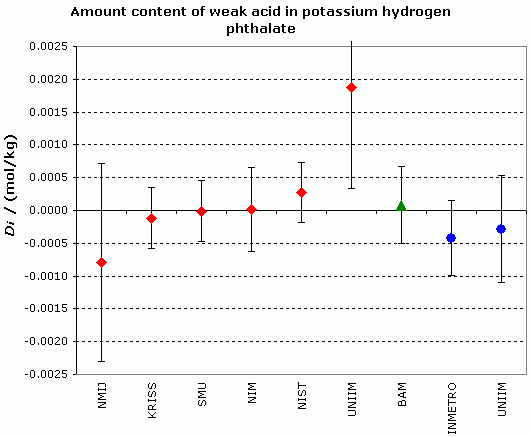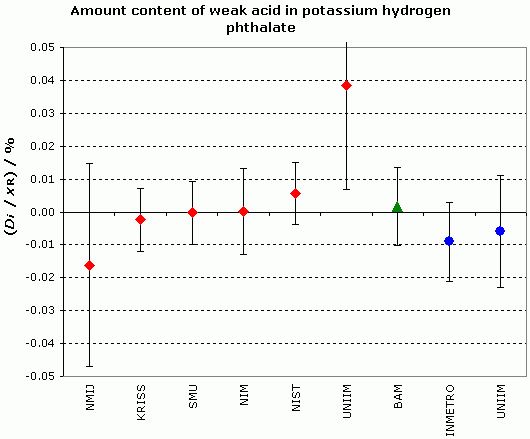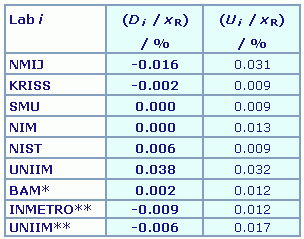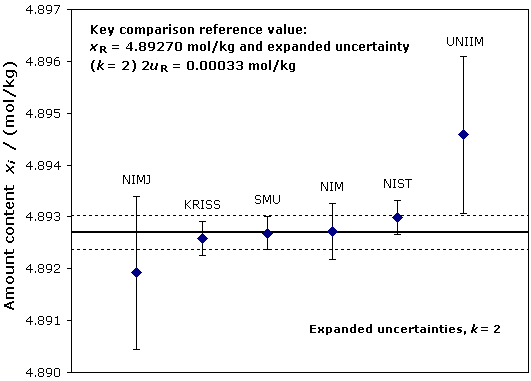Comparison
Comparison close
Results

CCQM-K34, CCQM-K34.1 and CCQM-K34.2

MEASURAND : Amount content of weak acid in potassium hydrogen phthalate
NOMINAL VALUE : ~ 4.897 mol/kg

Degrees of equivalence: Di = (xi - xR) and expanded uncertainty Ui (k = 2), both expressed in mol/kgDegrees of equivalence: (Di / xR) and expanded uncertainty (Ui / xR) (k = 2), both expressed in %Red diamonds: participants in CCQM-K34
Green triangle: participant in CCQM-K34.1
Blue circles: participants in CCQM-K34.2

Comparison
Comparison close
Results

CCQM-K34, CCQM-K34.1 and CCQM-K34.2

MEASURAND : Amount content of weak acid in potassium hydrogen phthalate
NOMINAL VALUE : ~ 4.897 mol/kg

 Lab i Di Ui / (mol/kg) / (mol/kg) NMIJ -0.0008 0.0015 KRISS -0.00012 0.00046 SMU -0.00001 0.00046 NIM 0.00001 0.00064 NIST 0.00028 0.00046 UNIIM 0.0019 0.0016 BAM* 0.0001 0.00058 INMETRO** -0.0004 0.00057 UNIIM** -0.0003 0.00082

* participant in CCQM-K34.1
** participants in CCQM-K34.2

Degrees of equivalence with respect to the key comparison reference value, expressed in relative terms: (Di / xR) and (Ui / xR) :* participant in CCQM-K34.1
** participants in CCQM-K34.2

Sets of absolute and relative degrees of equivalence are available in Summary Results (PDF file, presented under A4 printable format).

Comparison
Comparison close
CC comparison
 Metrology area, Sub-field Chemistry and Biology, Electrochemistry Description Assay of potassium hydrogen phthalate Time of measurements 2005 Status Approved for equivalence
 Final Reports of the comparisons Measurand Amount content, expressed in mol/kg Transfer device Dedicated samples
 Comparison type Key Comparison Consultative Committee CCQM (Consultative Committee for Amount of Substance) Conducted by CCQM (Consultative Committee for Amount of Substance) Comments This comparison is a follow-up of key comparison CCQM-K34.CCQM-K34.1 and CCQM-K34.2 results are linked to those of CCQM-K34 CCQM-K34.1 results published on 04 July 2007 Amount content of weak acid in potassium hydrogen phthalate Pilot institute SMU Slovensky Metrologicky Ustav Slovakia Contact person Michal Mariassy +421 2 602 94 522
First Name Last Name
wwww@ww.www +356719836 Institute 1 Institute 1 Khmelnitskiy
Pilot laboratory
SMU

Slovensky Metrologicky Ustav, Slovakia, EURAMET

 BAM Bundesanstalt für Materialforschung und -prüfung, Germany, EURAMET
Comparison
Comparison close
Results

CCQM-K34, CCQM-K34.1 and CCQM-K34.2

MEASURAND : Amount content of weak acid in potassium hydrogen phthalate
NOMINAL VALUE : ~ 4.897 mol/kg

• Key comparison CCQM-K34

 The key comparison reference value, xR, is calculated as the median of the participant results. Its standard uncertainty, uR, is based on the median of the absolute deviations, see on page 7 of the CCQM-K34 Final Report. xR = 4.89270 mol/kg, uR = 0.00016 mol/kg

 The degree of equivalence of laboratory i relative to the key comparison reference value is given by a pair of terms, both expressed in mol/kg:Di = (xi - xR) and its expanded uncertainty Ui (k = 2) where Ui = 2(ui2 + uR2)1/2.

 The degree of equivalence between two laboratories i and j is given by a pair of terms both expressed in mol/kg:Dij = (Di - Dj) = (xi - xj) and its expanded uncertainty Uij (k = 2) where Uij = 2(ui2 + uj2)1/2.
• Linking CCQM-K34.1 and CCQM-K34.2 to CCQM-K34

 SMU result is used as a link to the key comparison reference value from CCQM-K34. The results in the comparisons differ by less than 0.1 %, so the degrees of equivalence are directly comparable.

 The degree of equivalence of participant i with respect to the key comparison reference value is given by a pair of terms, both expressed in mol/kg:Di = (DSMU-K34 + xi-K34.a - xSMU-K34.a) and its expanded uncertainty Ui (k = 2) where Ui = 2(uSMU-K34.a2 + ui-K34.a2 + uR2)1/2, with a = 1 or 2.

 The degree of equivalence between BAM and laboratory j participant in CCQM-K34 is given by a pair of terms both expressed in mol/kg:DBAM, j = (DBAM - Dj) and its expanded uncertainty UBAM, j (k = 2) where UBAM, j = 2(uBAM-K34.12 + uSMU-K34.1 + uj2)1/2.Pair-wise degrees of equivalence involving CCQM-K34.2 participants are not calculated.
Comparison
Comparison close
Results

CCQM-K34, CCQM-K34.1 and CCQM-K34.2

• Key comparison CCQM-K34

MEASURAND : Amount content of weak acid in potassium hydrogen phthalate
NOMINAL VALUE : ~ 4.897 mol/kg

xi : result of measurement carried out by laboratory i
ui : combined standard uncertainty of xi

 Lab i xi / (mol/kg) ui/ (mol/kg) (ui / xi)/ % Date of measurement NMIJ 4.89192 0.00074 0.0151 Jun / Jul 2004 KRISS 4.89259 0.00016 0.0034 Aug 2004 SMU 4.89269 0.00016 0.0033 Jul 2004 NIM 4.89272 0.00027 0.0056 Jul / Aug 2004 NIST 4.89298 0.00016 0.0034 Jul 2004 UNIIM 4.89458 0.00076 0.0155 Aug 2004

Graph of laboratory individual measurements :
xi and expanded uncertainties (k = 2)• Key comparison CCQM-K34.1

MEASURAND : Amount content of weak acid in potassium hydrogen phthalate
NOMINAL VALUE : ~ 4.897 mol/kg

xi-K34.1 : result of measurement carried out by laboratory i participating in CCQM-K34.1
ui-K34.1 : combined standard uncertainty of xi-K34.1

 Lab i xi-K34.1 / (mol/kg) ui-K34.1/ (mol/kg) (ui-K34.1 / xi-K34.1)/ % Date of measurement BAM 4.89530 0.00016 0.0033 May / Jun 2005 SMU 4.89520 0.00018 0.0037 May / Jun 2005
• Key comparison CCQM-K34.2

MEASURAND : Amount content of weak acid in potassium hydrogen phthalate
NOMINAL VALUE : ~ 4.897 mol/kg

xi-K34.2 : result of measurement carried out by laboratory i participating in CCQM-K34.2
ui-K34.2 : combined standard uncertainty of xi-K34.2

 Lab i xi-K34.2 / (mol/kg) ui-K34.2/ (mol/kg) (ui-K34.2 / xi-K34.2)/ % Date of measurement INMETRO 4.89643 0.00018 0.0036 Oct 2008 UNIIM 4.89656 0.00034 0.0070 Dec 2008 SMU 4.89683 0.00015 0.0031 Oct 2008